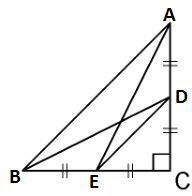Guru

# D and E are points on the sides CA and CB respectively of a triangle ABC right angled at C. Prove that AE2 + BD2 = AB2 + DE2.Q.13

• 0

Hello, sir please help me the best way to solve this problem of class 10th chapter of triangles chapter of exercise 6.5 of question no.13 give me the simple way to solve this problem, D and E are points on the sides CA and CB respectively of a triangle ABC right angled at C. Prove that AE2 + BD2 = AB2 + DE2.

Share

1. Given, D and E are points on the sides CA and CB respectively of a triangle ABC right angled at C.By Pythagoras theorem in ΔACE, we get

AC2 + CE2 = AE2 ………………………………………….(i)

In ΔBCD, by Pythagoras theorem, we get

BC2 + CD2 = BD2 ………………………………..(ii)

From equations (i) and (ii), we get,

AC2 + CE2 + BC2 + CD2 = AE2 + BD2 …………..(iii)

In ΔCDE, by Pythagoras theorem, we get

DE2 = CD2 + CE2

In ΔABC, by Pythagoras theorem, we get

AB2 = AC2 + CB2

Putting the above two values in equation (iii), we get

DE2 + AB2 = AE2 + BD2.

• 0